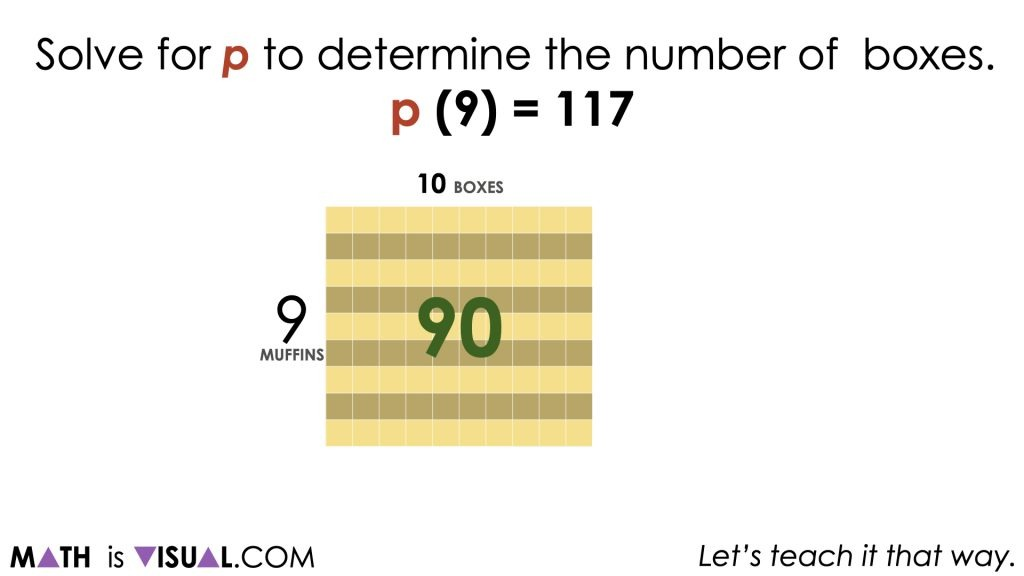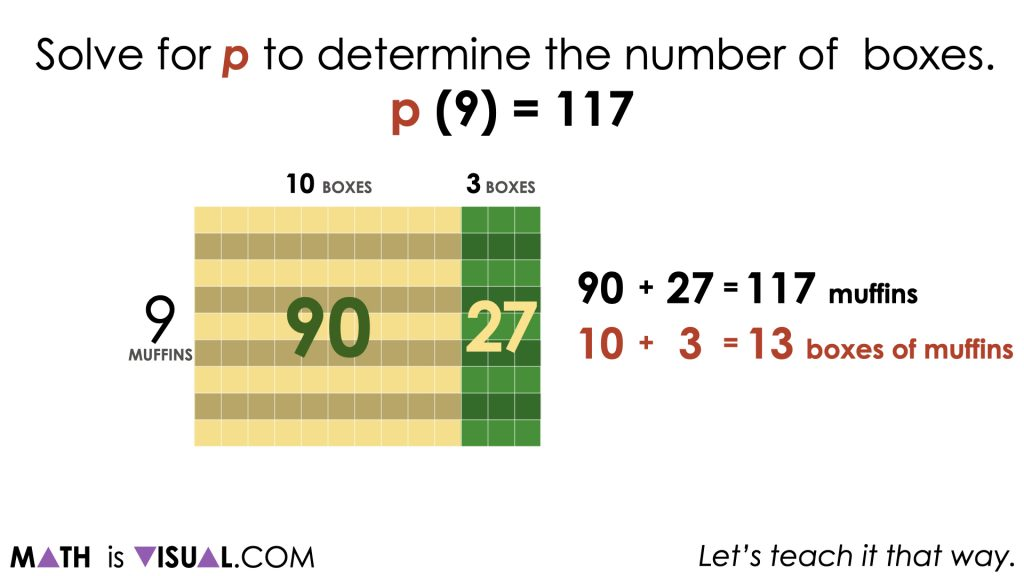# Solving One-Step Equations With Context

Visual math talk prompts with context used to help emerge the writing and solving of one-step algebraic equations.

## In This Set of Math Visual Prompts…

Students will explore quotative division problems with context and connect them to one-step algebraic equations.

## Intentionality…

This set of visual math talk prompts is taken from the Math Talk section of Day 5 in the Make Math Moments Problem Based Unit called Sowing Seeds Revisited. The purpose of the Math Talk is to reinforce key concepts and big ideas from this problem based math unit including:

• There are two types of division;
• Quotative division is when the total quota is known (the dividend), and the number per group or the rate (the divisor) is known;
• Quotative division reveals the number of copies or iterations of a rate that can be derived from the overall quota (the dividend);
• In quotative division, the dividend and the divisor have the same unit;
• The dividend from any division sentence can be decomposed into smaller parts to allow for friendlier division by the divisor. This strategy is known as partial quotients. (i.e.: 85 ÷ 5 = 45 ÷ 5 + 40 ÷ 5 = 9 + 8 = 17);
• Division is the inverse operation of multiplication;
• Variables are used to represent changing or unknown quantities;
• When solving an equation where the coefficient of the unknown variable is not equal to 1, division is required to determine the value of the unknown variable and the context of the problem determines which type of division is required.

Unsure about some of the terminology listed above?

## Preparing to Facilitate

In today’s visual math talk, students will use an appropriate model to solve the following one-step algebraic equations eliciting both partitive and quotative division. Encourage students to name the type of division required to solve and to create a context for each equation. Students are encouraged to use a model to solve and/or communicate their thinking.

p(9) = 117

Five (5) additional visual math prompts are shared in the Teacher Guide from Day 5 of the Sowing Seeds Revisited problem based math unit

Consider using the visual math talk prompts to help you prepare to facilitate this math talk or to assist you in doing so in a visual fashion. The purpose of this math talk is to reveal that equations using contexts that leverage both types of division can be solved using the same strategy and model.

Facilitator’s Note:

Take note that when the variable appears first, the variable is representing an unknown number of groups which will require quotative division. When the variable appears second, the variable is representing an unknown rate which will require partitive division.

## Visual Math Talk Prompt #1

In the video, students are presented with the following quotative division context:

p boxes of 9 muffins per box is equal to 117 muffins.

Solve for p to determine the number of boxes.

p(9) = 117

Although students are free to leverage the strategy and mathematical model of their choice, we are hoping to elicit the use of the open array or area model to demonstrate partial products and/or partial quotients.Students may choose to decompose the dividend in any way they would like, but a common practice would be to leverage partial products (i.e.: multiplying 9 muffins per box by 10 boxes) as a means to determine how to decompose and recompose the dividend of 117. In the visual above, you can see that since 10 boxes of 9 muffins per box results in 90 muffins, we now must make up for the remaining 27 muffins (117 – 90 = 27).Since we must account for the remaining 27 muffins, we will require an additional 3 boxes of muffins.

Therefore, if p(9) = 117, then p = 13 boxes.

## Want to Explore These Concepts & Skills Further?

Two (2) additional math talk prompts are available in Day 5 of the Sowing Seeds Revisited problem based math unit that you can dive into now.

Why not start from the beginning of this contextual 5-day unit of ratios and rates real world lessons from the Make Math Moments Problem Based Units page.

Did you use this in your classroom or at home? How’d it go? Post in the comments!

Math IS Visual. Let’s teach it that way.## Representing Ratios As PercentagesREGISTER FOR OUR FREE WEBINAR:
Reaching Every Student After The Toughest Year Ever
How to Focus On The Concept Holding Your Students Back

WATCH THE REPLAY NOW